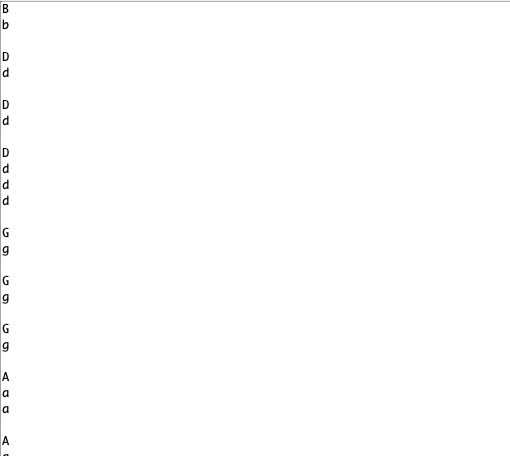# Problem with AT-09 bluetooth Arduino Connection

So i have made a 2WD Orangepip (like an arduino) bluetooth controlled car and i am having problems with the motors functioning.

The bluetooth does work as the serial monitor is printing the values i press on the joystick app. However, the motors are not working with the following codes. The motors do work in general as i did 3 other projects.

code:

#include <SoftwareSerial.h>

// Starting of Program
int MOT_A1_PIN = 5;
int MOT_A2_PIN = 6;
int MOT_B1_PIN = 9;
int MOT_B2_PIN = 10;
char val;

int leftServoSpeed = 0;
int rightServoSpeed = 0;

void setup()
{
pinMode(MOT_A1_PIN, OUTPUT);
pinMode(MOT_A2_PIN, OUTPUT);
pinMode(MOT_B1_PIN, OUTPUT);
pinMode(MOT_B2_PIN, OUTPUT);

digitalWrite(MOT_A1_PIN, LOW);
digitalWrite(MOT_A2_PIN, LOW);
digitalWrite(MOT_B1_PIN, LOW);
digitalWrite(MOT_B2_PIN, LOW);
Serial.begin(9600);
}

void set_motor_pwm(int pwm, int IN1_PIN, int IN2_PIN)
{
if (pwm < 0) { // reverse speeds
analogWrite(IN1_PIN, -pwm);
digitalWrite(IN2_PIN, LOW);

} else { // stop or forward
digitalWrite(IN1_PIN, LOW);
analogWrite(IN2_PIN, pwm);
}
}

void set_motor_currents(int pwm_A, int pwm_B)
{
set_motor_pwm(pwm_A, MOT_A1_PIN, MOT_A2_PIN);
set_motor_pwm(pwm_B, MOT_B1_PIN, MOT_B2_PIN);

}

void spin_and_wait(int pwm_A, int pwm_B, int duration)
{
set_motor_currents(pwm_A, pwm_B);
delay(duration);
}

void loop()
{
while (Serial.available() > 0)
{
Serial.println(val);
}

if( val == 'a' || 'A') // Forward
{
leftServoSpeed = 170;
rightServoSpeed = 140;

``````}
``````

else if(val == 'c' || 'C') // Backward
{
leftServoSpeed = -170;
rightServoSpeed = -140;
}

``````else if(val == 'd' || 'D') //Left
{

leftServoSpeed = 170;
rightServoSpeed = -140;

}

else if(val == 'b' || 'B') //Right
{
leftServoSpeed = -170;
rightServoSpeed = 140;
}
``````

else if(val == 'g' || 'G') //Stop
{
leftServoSpeed = 0;
rightServoSpeed = 0;
}
else if(val == 'a' && 'f' ) //Forward Right
{
leftServoSpeed = 170;
rightServoSpeed = 120;
}
else if(val == 'd' && 'f') //Backward Right
{
leftServoSpeed = -170;
rightServoSpeed = -120;
}
else if(val == 'a' && 'h') //Forward Left
{
leftServoSpeed = 120;
rightServoSpeed = 170;
}
else if(val == 'c' && 'h') //Backward Left
{
leftServoSpeed = -120;
rightServoSpeed = -170;
}

}Your compound logical conditionals with the "or" should be written like this

``````if( val == 'a' || val == 'A') //or conditional
``````

The conditionals with the "and" will never work as you constantly overwrite val, and won't ever get two values.

You will need to revise the sketch to read a complete message, and then operate on the command.

i have fixed what you pointed out and took out the commands with '&&' but it is still not sending it to the motors, just the serial monitor

code:

#include <SoftwareSerial.h>

// Starting of Program
int MOT_A1_PIN = 5;
int MOT_A2_PIN = 6;
int MOT_B1_PIN = 9;
int MOT_B2_PIN = 10;
char val;

int leftServoSpeed = 0;
int rightServoSpeed = 0;

void setup()
{
pinMode(MOT_A1_PIN, OUTPUT);
pinMode(MOT_A2_PIN, OUTPUT);
pinMode(MOT_B1_PIN, OUTPUT);
pinMode(MOT_B2_PIN, OUTPUT);

digitalWrite(MOT_A1_PIN, LOW);
digitalWrite(MOT_A2_PIN, LOW);
digitalWrite(MOT_B1_PIN, LOW);
digitalWrite(MOT_B2_PIN, LOW);
Serial.begin(9600);
}

void set_motor_pwm(int pwm, int IN1_PIN, int IN2_PIN)
{
if (pwm < 0) { // reverse speeds
analogWrite(IN1_PIN, -pwm);
digitalWrite(IN2_PIN, LOW);

} else { // stop or forward
digitalWrite(IN1_PIN, LOW);
analogWrite(IN2_PIN, pwm);
}
}

void set_motor_currents(int pwm_A, int pwm_B)
{
set_motor_pwm(pwm_A, MOT_A1_PIN, MOT_A2_PIN);
set_motor_pwm(pwm_B, MOT_B1_PIN, MOT_B2_PIN);

}

void spin_and_wait(int pwm_A, int pwm_B, int duration)
{
set_motor_currents(pwm_A, pwm_B);
delay(duration);
}

void loop()
{
while (Serial.available() > 0)
{
Serial.println(val);
}

if( val == 'a' || val == 'A') // Forward
{
leftServoSpeed = 170;
rightServoSpeed = 140;

``````}
``````

else if(val == 'c' || val == 'C') // Backward
{
leftServoSpeed = -170;
rightServoSpeed = -140;
}

``````else if(val == 'd' || val == 'D') //Left
{

leftServoSpeed = 170;
rightServoSpeed = -140;

}

else if(val == 'b' || val == 'B') //Right
{
leftServoSpeed = -170;
rightServoSpeed = 140;
}
``````

else if( val == 'G') //Stop
{
leftServoSpeed = 0;
rightServoSpeed = 0;
}

}

I have no idea if your three motor functions work properly, but you never call them and pass them the servo speed values you set with commands over bluetooth.

You need to start simple and build up from small things which work. Get one motor to run in one direction when bluetooth reads 'a' and to stop when you read 'g'.

thank you it is working now, i took off the servo speed functions and just made digitalWrite(output,high) and lows for the directions. Thank you for your help, was very much appreciated

This topic was automatically closed 120 days after the last reply. New replies are no longer allowed.